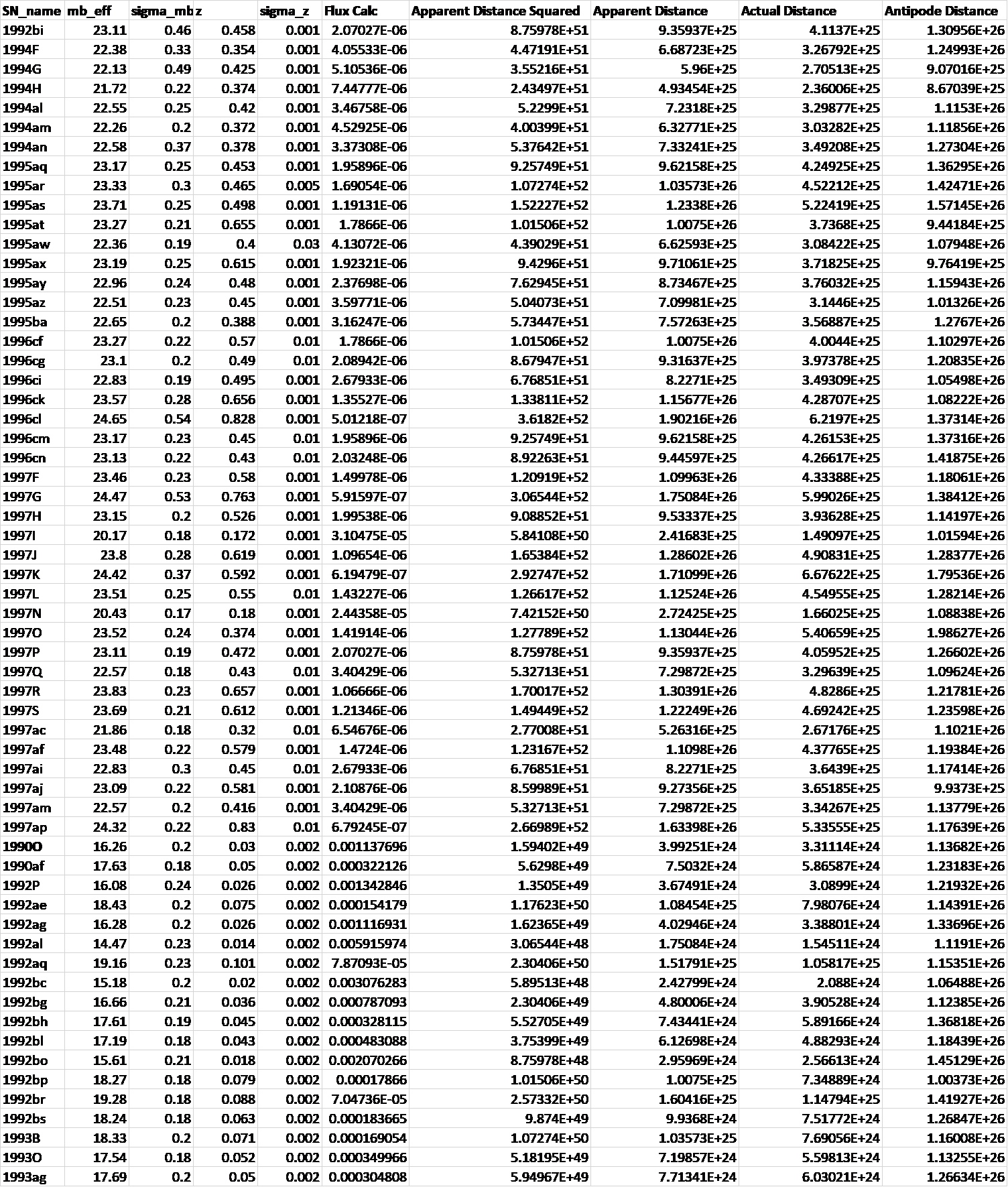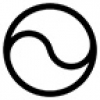## Hypersphere CosmologyAlternative to big-bang

Tuesday, 17 December 2019 21:15

## Type 1A supernovae and Hypersphere Cosmology

Lensing, Redshift and Distance for Type1A Supernovae.

All data input from Perlmutter et all, https://arxiv.org/pdf/astro-ph/9812133.pdf

The measured Apparent Magnitudes of type 1A Supernovae become converted into fluxes measured in Janskys and these fluxes become converted into Apparent Distances on the basis of the inverse square law taking the Absolute Magnitude of a type 1A supernova as -19.3 at 10 parsecs as the basis of calculation.

The Lensing Equation  then gives the Actual Distance for each supernova.

The Redshift-Distance Equation  then gives the Antipode distance of the Universe which comes out at ~13 billion light years with only minor divergences, in every case.

The physical principles underlying the Lensing Equation and the Redshift-Distance Equation lie here https://www.specularium.org/hypersphere-cosmology in sections 8 and 5 respectively.

This analysis suggests that the small positive spacetime curvature of a Hyperspherical Universe can account for the apparent discrepancies between observed redshifts and observed apparent magnitudes of type 1A, and the hypotheses of an expanding universe with an accelerating expansion driven by a mysterious ‘dark energy’ become unnecessary.• #### HyperSphere Cosmology +Hypersphere Cosmology in a Nutshell Abstract. Hypersphere Cosmology presents an alternative to the expanding universe of the standard LCDM Big
• #### Hypersphere Redshift +The alternative mechanism for redshift works as follows: Redshift = Z =   - 1 Where = observed wavelength, = expected wavelength, and the -1
• #### Hypersphere Rotation +The Gödel 3d rotating universe has this solution derived from General Relativity: - “Matter everywhere rotates relative to the compass of
• #### Hypersphere Holometry - A Prediction +As a consequence of the hypothesis that the universe exists as a hypersphere where   the main parameters of the
• #### VHC. Vorticitating Hypersphere Cosmology +The overwhelming majority of university and state funded cosmologists believe that the observable universe has expanded from a much smaller
• #### Hypersphere Visualisation & Lensing +This paper provides a method of visualising the four dimensional hypersphere as a perspective construction in three dimensions, and it
• #### Sacred Geometry +

As faith began to make way for reason during the Enlightenment, humans began to deduce the relationships between certain mathematical
• #### Starships +

Houston we have a problem. If the human race does not develop starships that can reach other star systems as
• #### Quantum & Probability +

I have repeatedly asserted in many of my magical and scientific books and papers that this universe runs on probability
• #### Ur Chaos Theory +

In this paper 'Chaos' denotes randomness and indeterminacy, not the mere so-called 'deterministic chaos' of systems with extreme sensitivity to
• #### Type 1A supernovae and Hypersphere Cosmology +

Lensing, Redshift and Distance for Type1A Supernovae. All data input from Perlmutter et all, https://arxiv.org/pdf/astro-ph/9812133.pdf The measured Apparent Magnitudes of

• 1